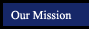Line Segments Triangles Parallel and Perpendicular Lines Construct a congruent line segment Construct a congruent triangle given three side lengths Construct a parallel line through a point not on the line Construct a line segment twice the length of a given segment Construct an equilateral triangle Construct a Perpendicular Bisector Construct a right isosceles triangle Construct a perpendicular line through a point on the line Angles Construct a triangle given two sides and the included angle (SAS) Construct a perpendicular line through a point not on the line Construct a congruent angle (acute example) Construct a triangle given two angles and the included side (ASA) Polygons Construct a congruent angle (obtuse example) Construct a circle inscribed in a triangle Construct a regular hexagon Construct an angle bisector Construct a triangle circumscribed by a circle Construct a rectangle Construct a right angle Construct a 45 degree angle Construct a 60 degree angle Construct a 30 degree angle